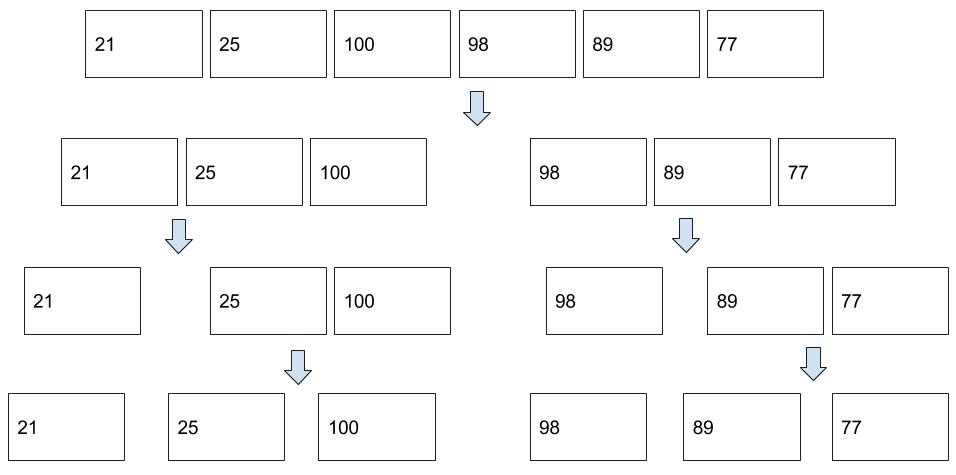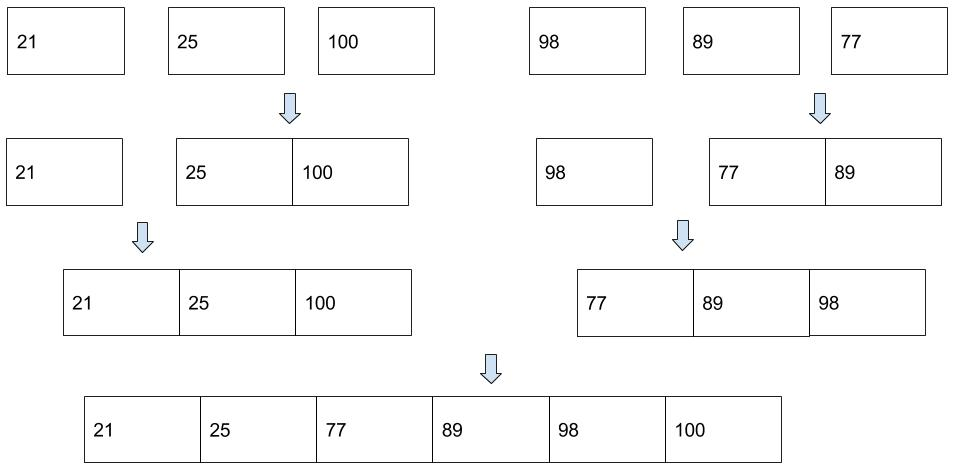# #Data Structure and Algorithm - Merge Sort

In this tutorial, we will learn a simple sorting algorithm - Merge Sort.

## #Problem to Solve

Given a list of numbers as shown below, please sort them in ascending order.

``````\$numbers = [21,25,100,98,89,77];
``````

Requirements:

• You are required to use Merge Sort algorithm when sorting the numbers.
• You are required to implement the algorithm in PHP language.

## #Pseudocode

Merge Sort is a divide and conquer algorithm. It works by continually splitting a list in half until both halves are sorted, then the operation merge is performed to combine two lists into one sorted new list.

When splitting a list, we consider the list is sorted if it contains zero or one element.

Split:Merge:Pseudocode of Merge Sort algorithm can be written as follows:

``````PROCEDURE function mergeSort
FOR each element of the master list indexed by i

if ( i <= 1 ) return a

var left = a to a[i/2]
var right = a[i/2+1] to a[i]

left = mergeSort( left )
right = mergeSort( right )

return merge( left,right )

END FOR
END PROCEDURE

PROCEDURE function mergeSort
WHILE length(left) > 0 and length(right) > 0
if first(left) â‰¤ first(right)
append first(left) to result
left = rest(left)
else
append first(right) to result
right = rest(right)

IF length(left) > 0
append left to result
END IF

IF length(right) > 0
append right to result
END IF

return result
END PROCEDURE
``````

## #PHP Implementation

As we can see, there are two procedures in this algorithm. This leads us to two PHP functions, where the first function(mergeSort) involves a recursion.

``````<?php
\$arrayToSort = [21, 25, 100, 98, 89, 77];

function mergeSort(\$data)
{
if (count(\$data) <= 1) {
return \$data;
}

\$left = array_slice(\$data, 0, intval((count(\$data) / 2)));
\$right = array_slice(\$data, intval(count(\$data) / 2));

\$left = mergeSort(\$left);
\$right = mergeSort(\$right);

return merge(\$left, \$right);
}

function merge(\$left, \$right)
{
\$sorted = [];

while (count(\$left) > 0 && count(\$right) > 0) {
if (\$left < \$right) {
\$firstLeft = array_shift(\$left);
\$sorted[] = \$firstLeft;
} else {
\$firstRight = array_shift(\$right);
\$sorted[] = \$firstRight;
}
}

for (\$i = 0; \$i < count(\$left); \$i++)
\$sorted[] = \$left[\$i];

for (\$i = 0; \$i < count(\$right); \$i++)
\$sorted[] = \$right[\$i];

return \$sorted;
}

print_r(mergeSort(\$arrayToSort));

// Output:
/*
Array
(
 => 21
 => 25
 => 77
 => 89
 => 98
 => 100
)
*/
``````

We have followed the pseudocode strictly to implement the algorithm in PHP. The only parts that we want to highlight are a couple of built-in PHP array functions:

• array_slice: It extracts a slice of the array. This function is handy when we want to a certain part of the array.
• array_shift: It remove an element from the beginning of an array. This function is handy when we want to remove the first element of the array.